# SAT Math Multiple Choice Question 767: Answer and Explanation

### Test Information

Question: 767

2.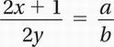If a and b are non-zero constants in the linear equation above, what is the slope of this line when it is graphed in the xy-plane?

• A.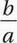• B.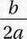• C.• D.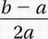Explanation:

A

Algebra (linear equations) MEDIUM-HARD

Perhaps the best way to find the slope of the line is to get the equation into "slope-intercept form" (Chapter 7, Lesson 5).

Original equation: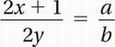Cross-multiply:

a(2y) = b(2x + 1)

Simplify:

2ay = 2bx + b

Divide by 2a: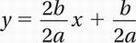Simplify: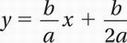Therefore, the slope of the line is b/a.